# Type of SemiConductors

Semiconductors are classified into Intrinsic semiconductors and Extrinsic semiconductors.
Extrinsic semiconductor further may be classified into N-type and P-type semiconductor.

### Intrinsic semiconductor

A semiconductor is an extremely pure for is known as an intrinsic semiconductor.
Common examples of such semiconductor are: pure Germanium and silicon which have forbidden energy gaps of 0.72 eV and 1.1 eV respectively.

Alternatively an intrinsic semiconductor may be desired as one in which the number of conduction electrons is equivalent to the integer of holes.

In an intrinsic semi-conductor, uniform at room temperature, hole % electron pairs are produced when electric field is applied through an intrinsic semi-conductor, the current conduction proceeds by 2 process, that is; by free electrons and holes as shown in the figure.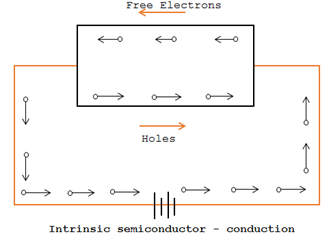Schematic energy bond illustration of an intrinsic semi-conductor at room temperature is shown below: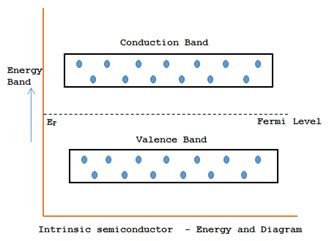The free electrons are created due to the breaking up of various covalent bonds by thermal energy. Similarly, holes are produced in the covalent bonds. Under the effect of electric field, conduction over the semi-conductor by both free electrons is holes. Hence, the entire current inside the semi-conductor is the sum of currents owing to free electrons and holes.

### Extrinsic Semi-conductors

By adding a minor amount of appropriate impurity (Donor) to an intrinsic semi-conductor, it converts an extrinsic semiconductor.
Intrinsic semi-conductors are so-called extrinsic semiconductors on which specific suitable impurity or doping agent has been added in exceptionally lesser amount.

#### Doping

It’s the process of adding impurities to the semiconductors. The amount and types of such impurities have to be carefully controlled for the period of the preparation of extrinsic semi-conductor.
The determination of increasing impurity is to raise either the amount of free electrons or holes in the semi-conductor crystal.
Reliant upon the kinds of impurity added extrinsic semi-conductors are classified into:
1. N-type semi-conductors
2. P-type semi-conductors

### N-Type semi-conductors

When a lesser amount of pentavalent impurities are added to a pure semi-conductor, it is named as n-type semiconductor. (N stands for Negative).
The adding of pentavalent impurity delivers a huge number of free electrons in the semi-conductor crystal.
Usual example of pentavalent impurities is arsenic (AS) and antimony (Sb). Such impurities which create n-type semi-conductors are known as donor impurities since they donate or deliver free electrons to the semi-conductor crystal.

#### Creation of N-Type semiconductor

It is obtained when a pentavalent material like antimony is added to pure geranium crystal. As shown in figure each antimony atoms forms covalent bonds with the surrounding four geranium atoms with the help of four of its five electrons.
Practically every antimony atom introduced into the germanium lattice contributes one conduction electron without creating a positive hole.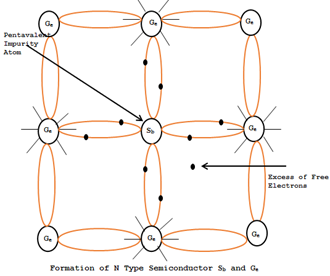The figure shows the energy band description of n-type semi-conductors. The addition of pentavalent impurities has formed a number of conduction-band electrons. i.e. free electrons

###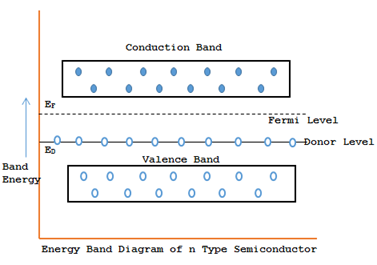P-Type Intrinsic Semiconductors:

When a minor amount of trivalent impurities are added to a pure semiconductor, it is named as P-type semiconductor. (P stands for Positive).
The additions of trivalent impurity provide a great number of holes in the semi-conductor.
Usual examples of trivalent impurities are Gallium (Ga), Induim(Id) and Boron(B). Such impurities which create P-type semi-conductor are known as acceptor impurities since the holes created can receive the electrons.
This kind of semi-conductor is acquired when traces of a trivalent impurity like boron(B) are added to a pure germanium crystal.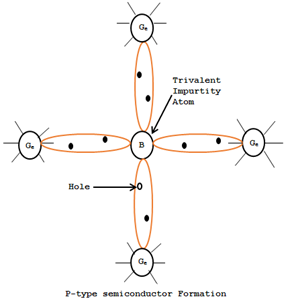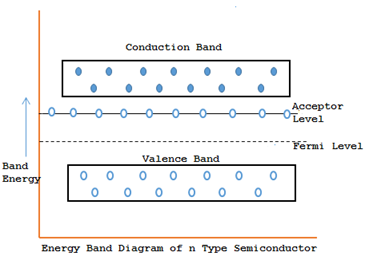Therefore boron which is called an acceptor impurity causes a lot of positive holes in a germanium crystal as there are boron atoms there by providing a P-type extrinsic semi-conductor.
The above figure shows the energy band description of the P-type semi-conductor. The addition if trivalent impurity has formed a large number id holes. But, there are a little conduction band electrons due to thermal energy allied with room temperature. But the holes for outnumber the conduction band electrons.0 CommentsComments